# Op Amp Combines Femtoamp Bias Current with 4GHz Gain Bandwidth Product, Shines New Light on Photonics Applications

Einstein published his seminal paper on the photoelectric effect 110 years ago, essentially inventing the discipline of photonics. One would think that over so many years the science and engineering surrounding photonics must have fully matured. But not so. Optical sensors—photodiodes, avalanche photodiodes, and photomultiplier tubes—continue to achieve astoundingly high dynamic ranges, enabling electronics to peer ever more deeply into the photonic world.

Photosensors typically convert photons to electron current and are followed by a transimpedance function to transform the current into a voltage. The transimpedance function may be either a simple resistor or, for higher bandwidth, the summing node of an op amp, in which case it is called a transimpedance amplifier (TIA). The traditional enemies of the TIA are voltage noise, current noise, input capacitance, bias current and finite bandwidth. Enter the new LTC6268-10 with 4.25nV/√Hz voltage noise, 0.005pA/√Hz current noise, a very low 0.45pF of input capacitance, 3fA of bias current and 4GHz of gain-bandwidth.The LTC6268’s performance meets the demands of the latest photonics applications.

Understanding Voltage Noise And Current Noise Contributions In TIAs

Output noise in TIAs is a result of combined input voltage noise and input current noise. This combined effect is often specified as a current noise referred to the input—essentially the output voltage noise divided by the gain in ohms—but it actually arises from both input noise sources. In fact, the dominant cause of output noise is usually input voltage noise (Figure 1).Figure 1. The op amp with its noise sources and input capacitance. Total op amp noise (ignoring RF thermal noise) is INOISE = in + 2πfCINen (added rms-wise).

By virtue of feedback, the minus input is fixed at virtual ground so the current noise in passes directly through RF and contributes to total current noise with a factor of 1. Also by virtue of feedback, the voltage noise en is placed in parallel with the input capacitance CIN and induces a current noise of en/Z(CIN). The impedance of a capacitor is 1/2πfC, so the effective current noise due to input voltage noise and capacitance is 2πfCINen. So the total op amp noise (ignoring RF thermal noise) is

This is sometimes referred to as CV + I noise and makes an excellent figure of merit for an op amp, because it incorporates only op amp characteristics, neglecting external aspects of the circuit such as photosensor capacitance and RF thermal noise. It is essentially the best the op amp can do.

Sample Calculation And Comparison Between LTC6268-10 And Competitive OPA657

The CV + I noise is a useful figure of merit for comparing op amps, but it does have a dependency on frequency. An insightful comparison can be made by initially comparing them at a specific frequency and then observing the differences in the plots of CV + I noise versus frequency that inevitably arise. For example, let’s compare the LTC6268-10 and competitive OPA657 by starting with a calculation at 1MHz.

The LTC6268-10 data sheet gives plots of current noise versus frequency showing 0.05pA/√Hz at 1MHz, and of voltage noise versus frequency showing 4nV/√Hz at 1MHz. Using the input capacitance of 0.55pF (0.45pF for CCM, plus 0.1pF for CDM), the total CV noise at 1MHz can be calculated as

Summing this rms-wise with the native I noise of 0.05pA/√Hz, we get 0.052pA/√Hz of total CV + I noise at 1MHz.

The same calculation for the competitive OPA657 can also be performed. It specifies 4.8nV/√Hz voltage noise, 5.2pF input capacitance (4.5pF for CCM plus 0.7pF for CDM), and 1.3fA/√Hz current noise. Calculating total CV + I noise gives 0.156pA/√Hz at 1MHz for the OPA657, about three times worse than LTC6268-10.

Figure 2 shows a plot of CV + I noise for LTC6268-10 and OPA657 versus frequency. The reason the LTC6268-10 outperforms the OPA657 is its lower voltage noise and its much lower input capacitance. And because the LTC6268-10 has lower voltage noise, it continues to outperform the OPA657 as the sensor capacitance is added and increased. Furthermore, the LTC6268-10 features a rail-to-rail output and can operate on a single 5V supply, burning half the power of OPA657.Figure 2. CV + I current noise versus frequency for the LTC6268-10 and OPA657. The LTC6268-10 is considerably quieter.

Gain Bandwidth, And Achieving High Bandwidth At High Impedance

Another advantage of the LTC6268-10 is its serious 4GHz gain bandwidth product. In fact, you’ll find that the LTC6268-10 is able to find and use tiny parasitic capacitances that other op amps miss. Normally, high value resistors begin to reduce their net impedance at high frequency due to their end-to-end capacitance. The key to exploiting the 4GHz gain bandwidth of the LTC6268-10 with higher gain TIAs is to minimize the feedback capacitance around the main feedback resistor. Though minimized, the LTC6268-10 can use the tiny residue feedback capacitance to compensate the feedback loop, extending resistor bandwidth to several MHz. Following is a design example at 402k.

Good layout practices are essential to achieving best results from a TIA circuit. The following two examples show drastically different results from an LTC6268-10 in a 402k TIA (Figure 3). The first example is with an 0805 resistor in a basic circuit layout. In a simple layout, without expending a lot of effort to reduce feedback capacitance, the rise time achieved is about 88ns (Figure 4), implying a bandwidth of 4MHz (BW = 0.35/tR). In this case, the bandwidth of the TIA is limited not by the GBW of the LTC6268-10, but rather by the fact that the feedback capacitance is reducing the actual feedback impedance (the TIA gain itself) of the TIA. Basically, it’s a resistor bandwidth limitation. The impedance of the 402k is reduced by its own parasitic capacitance at high frequency. From the 4MHz bandwidth and the 402k low frequency gain, we can estimate the total feedback capacitance as

That’s fairly low, but it can be reduced further, maybe much further.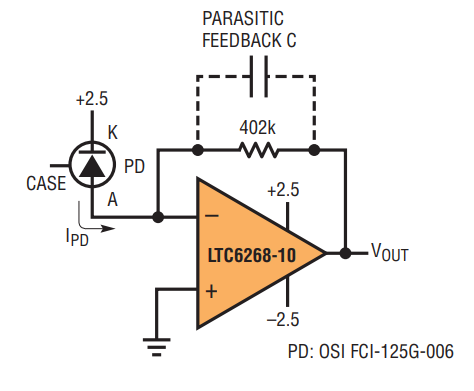Figure 3. LTC6268-10 and low capacitance photodiode in a 402kΩ TIA.Figure 4. Time domain response of 402kΩ TIA without extra effort to reduce feedback capacitance. Rise time Is 88ns and BW is 4MHz.

With some extra layout techniques to reduce feedback capacitance, the bandwidth can be increased. Note that we are increasing the effective “bandwidth” of the 402k resistance. A very powerful method to reduce feedback capacitance is to shield the E field paths that give rise to the capacitance. In this case, the method is to place a ground trace between the resistor pads. Such a ground trace shields the output field from getting to the summing node end of the resistor, effectively shunting the field to ground instead. The trace increases the output load capacitance very slightly. See Figure 5a and 5b for a pictorial representation, and Figure 5c for an example layout.Figure 5. A normal layout (a) and a fieldshunting layout (b). Circuit board in (c) shows actual layout with extra shunting at R9, less at R12. Simply adding a ground trace under the feedback resistor does much to shunt field away from the feedback side, dumping it to ground. Note that the dielectric constant of FR4 and ceramic is typically 5, so most of the capacitance is in the solids and not through the air. Such field shunting techniques reduced feedback capacitance from approximately 100fF in Figure 4 to 11.6fF in Figure 6. Note also that the feedback trace is exposed in upper (c) but entirely shielded in lower (c).

Figure 6 shows the dramatic increase in bandwidth simply by careful attention to low capacitance methods around the feedback resistance. Bandwidth and rise time went from 4MHz (88ns) to 34MHz (10.3ns), a factor of 8. The ground shield trace used for the LTC6268-10 was much wider than that used in the high speed case of the LTC6268 (see LTC6268 data sheet), extending under the entire resistor dielectric. Assuming all the bandwidth limit is due to feedback capacitance (which isn’t fair), we can calculate an upper limit ofFigure 6. LTC6268-10 in a 402kΩ TIA with extra layout effort to reduce feedback capacitance achieves 10.3ns total system rise time, or 34MHz total system bandwidth. This is an 8x increase in bandwidth, due to a well placed bit of ground trace.

A Photomultiplier Tube (PMT) At Lower Impedance

Photomultiplier tubes (photograph and x-ray shown in Figure 7) yield photonics gains above one million, meriting their considerably high cost. Given the high inherent gain, the TIA gain can be reduced, and bandwidth extended to the point that single photon events can be isolated. One convenient feature of a PMT is self-excitation, drawing energy either from local cosmic radiation or its own thermionic electron emission when the plate voltage is high, producing a random Dirac-deltalike ping of electrons on the output plate.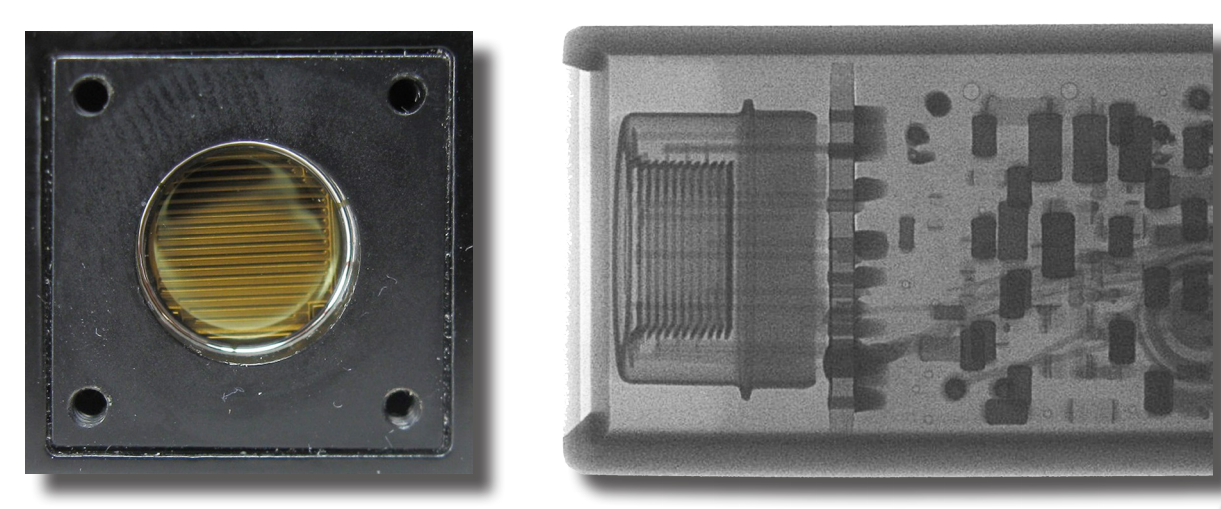Figure 7. A photograph and x-ray of a Hamamatsu photomultiplier tube. The electronics components visible at right are the encapsulated high voltage supply. (Do not x-ray your PMT unless it is already unusable.)

When using the LTC6268-10 at low gain, however, care must be taken to ensure its gain stability requirement of 10 is met, or there is risk of oscillation. The Hamamatsu PMT did not have a specified output plate capacitance, but the HP4192 impedance analyzer measured it to be 10pF at its maximum test frequency of 13MHz. Given that fact, a feedback capacitance of 1pF should have been adequate to ensure an apparent noise gain of 11.

However, the pins on the PMT were about 3/4 inch long (Figure 8), and with the LTC6268-10 connected to it in a gain of 1.82k, a sustained oscillation of 1.05GHz became apparent alongside the expected response to a dark-current ping (Figure 9). Trying a variety of feedback capacitors between 0.2pF and 1pF around the LTC6268-10 did not help. The conclusion was that the short transmission line was changing the appearance of the 10pF plate at high frequency, and was therefore not satisfying the gain of 10 requirement.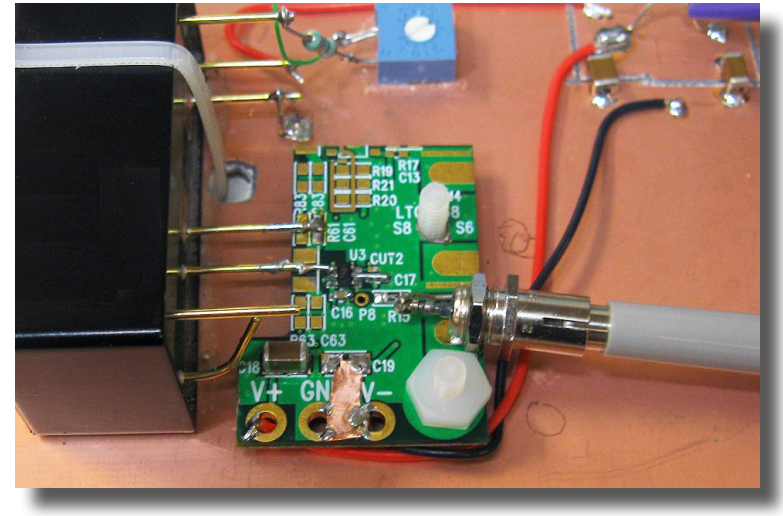Figure 8. First attempt at connecting LTC6268-10 to PMT output plate. Note the 3/4-inch or so transmission line created by the PMT plate pin. That’s far below 1/4-lambda at 300MHz. What could possibly go wrong? See Figure 9.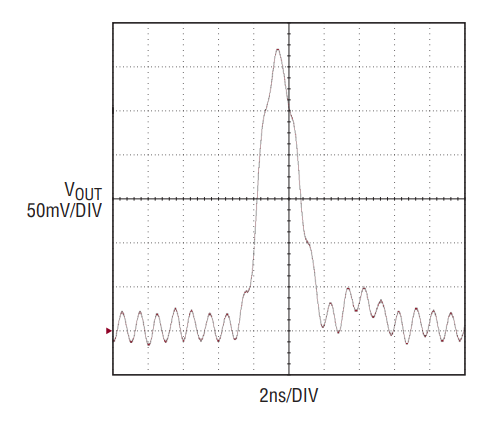Figure 9. The transmission line was short compared to a 300MHz assessment, but long enough to be a problem when compared to real bandwidth available.

With the LTC6268-10 positioned closer to the PMT body on a new board (Figure 10), the oscillation was quenched and the much improved response of Figure 11 was achieved. Component feedback capacitance installed was 0.8pF (Murata GJM1555C1HR80). Another change on the board was that the feedback resistor was brought to the topside, eliminating two vias.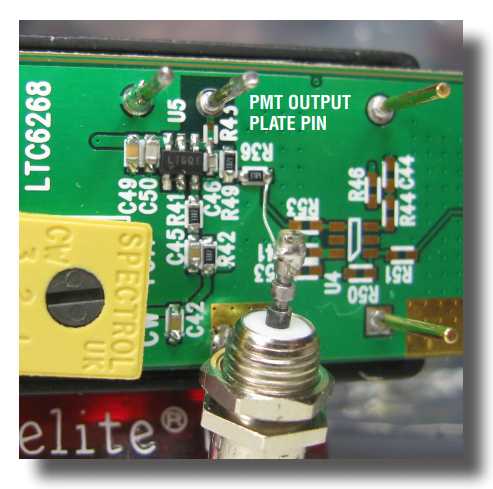Figure 10. Much tighter design on dedicated board. LTC6268-10 is now much closer to the PMT body and therefore the PMT output plate capacitance. Transmission line still exists, but it is hanging midair and is not “in the way.” .Figure 11. Reducing the transmission line length is key to achieveing good results. Output pulse half-width is 2.2ns. Exact −3dB bandwidth is not as relevant as a clean timedomain response.

Measuring Femtoamps

The LTC6268 achieves bias currents about two orders of magnitude lower than any previous Linear Technology amplifier, which requires accurately measuring femtoamps—while measuring picoamps is challenging enough. In production testing, speed is of the essence, so capacitive switching techniques are employed. In our tests made on the bench, where speed is not an issue, a sense resistor was preferred.

Assuming a 1mV op amp offset allowance (actually 0.7mV max), and a desired resolution of 1fA, the required sense resistor comes to 1mV/1fA = 1TΩ. Fortunately, Ohmite makes a 1T resistor, in the long blue MOX1125 package. In order to measure input bias current at various input common mode voltrage levels to the DUT (device under test), the circuit of Figure 12 was employed.Figure 12. Circuit for measuring femtoamp bias current of LTC6268 (the unity gain stable version of LTC6268-10) and measured results, at various common modes.

Circuit board effects were removed by removing the circuit board. That is, removing the board under the LTC6268 noninverting input and whisker connecting it through air to the 1TΩ resistor. This leaves just the op amp pin, the resistor and their package materials in place, hanging midair, as you can see in Figures 13 (topside) and 14 (bottom side).Figure 13. Actual board implementation of the femtoamp measurement board. Note the placement respecting the long blue resistor. Feedback capacitance to DUT input pin is through-air only.Figure 14. The bottom side of the board, showing the DUT input pin hanging midair.

Figure 15 shows the time domain response, settling well in 2.2 seconds. The overshoot isn’t actually overshoot in the conventional sense, but rather the charge necessary to move the total input C, effectively looking like a short term bias current. The voltage delta of the overshoot is about 190mV, and extends about 1.25 seconds in width. The total charge can be estimated by calculating the area of the triangle created by the voltage-overshoot in Figure 15: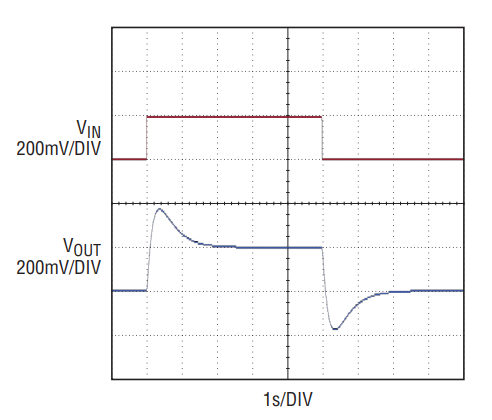Figure 15. Time domain response. Settles in 2.2 seconds with a 200mV change in common mode voltage. Overshoot is real, as the teraohm resistor moves the voltage on the 0.6pF total input capacitance.

With Q = CV, and a 200mV step, the total input C can be calculated as Q/V = 0.6pF. A rough allocation would be 0.45pF for the LTC6268 input CDM and another 0.15pF for the whisker and resistor lead. Output noise was measured at just under 1mVP–P, consistent with the objective of resolving 1fA.

Conclusion

The LTC6268-10 significantly reduces the traditional enemies of TIAs: voltage noise, current noise, input capacitance and bias current. It features extremely low 4.25nV/√Hz voltage noise, 0.005pA/√Hz current noise, a very low 0.43pF of input capacitance, 3fA of bias current and 4GHz of gain-bandwidth.